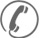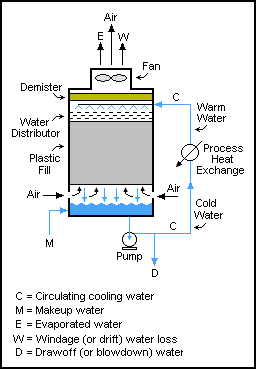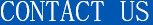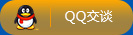18003967999

# 湿式蒸发冷却塔系统周围的物料平衡m =补给水，单位m3/h

c =循环水，单位m3/h

d =抽水量m3/h

e =蒸发水m3/h

w =水的风压损失，单位为m3/h

ppmw =百万分之一重量

M = E + D + W

{\ displaystyle MX_ {M} = DX_ {C} + WX_ {C} =间{C} (D + W)} M X_M = D X_C + W X_C = X_C (D + W)

,因此:

{\ displaystyle{间C{} \ /间{M}} ={\文本{的循环浓度}}= {M \ / (D + W)} = {M \ / (E)} = 1 + {E \ / (D + W)}} {X_C \ / X_M} = \文本{的循环浓度}= {M \ / (D + W)} = {M \ / (M - E)} = 1 + {E \ / (D + W)}

{\displaystyle E={C\Delta Tc_{p} \over H_{V}} E={C\Delta T c_p \over H_V}

hv =水汽化潜热= 2260 kJ / kg

Δt =水温差从塔上塔下,°C

cp =水比热= 4.184 kJ / (kg {\displaystyle \cdot} \cdot℃)

W = 0。3%到1。0%的C，用于没有风移消除器的自然通风冷却塔

W = 0。1%到0。3%的C，用于没有风移消除器的诱导通风冷却塔

W =约0.0005%的C(或更少)，如果冷却塔有风移消除器，并使用海水作为补充水。

## 公司产品###